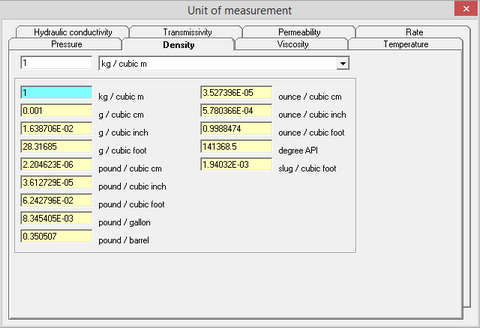﻿ Help > Unit converter (Part 1) > Density

# Density

Navigation:  Help > Unit converter (Part 1) >

# Density

Density is a measure of mass per volume. The average density of an object equals its total mass divided by its total volume. Density is measured in kg/m3 (SI system) and in g/cm3 (SGS system).Tab "Density" of the dialog window "Unit converter (part 1)"

In the petroleum industry API (American Petroleum Institute) units are widely used for oil density measurements. The API density is calculated in degrees by the formula:

°API = (141.5/relative density) - 131.5

Relative density - is the mass of volume of specific liquid/substance divided by the mass of the same volume of water. For clean water, relative density equals to 1 and API equals to 10°. The higher the density is in °АРI, the lighter the substance is.